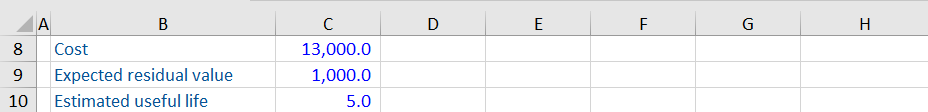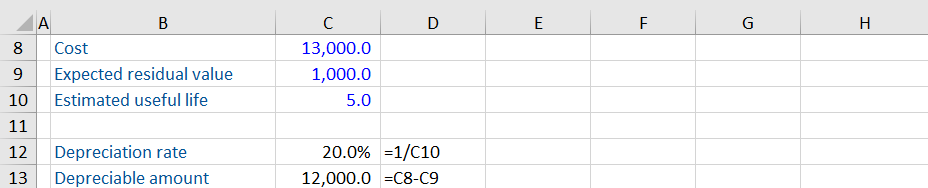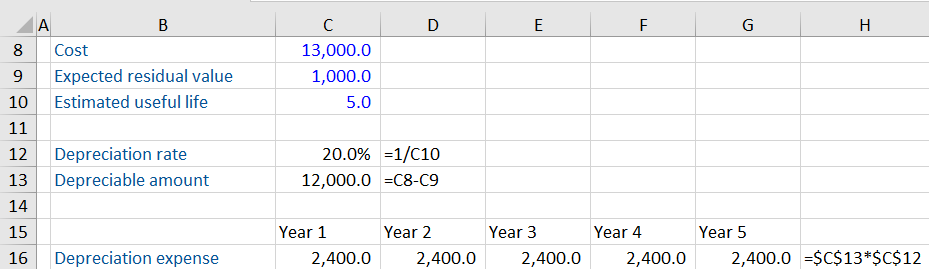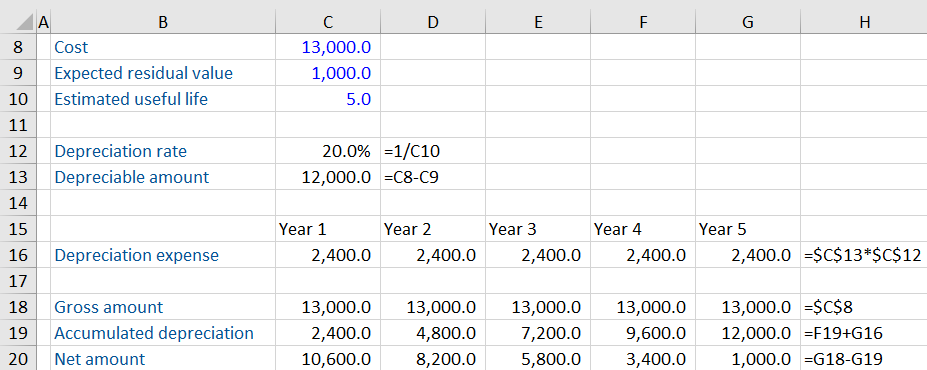## What is Straight Line Depreciation?

Straight line depreciation is a common cost allocation method which expenses the same depreciation charge for each year of a long-term tangible asset’s useful life. The future benefits of the asset are expensed at the same rate each year.

Depreciation is a non-cash charge expensed through the income statement. The net book value of the asset decreases by the same amount as the charge for the year.

## Key Learning Points

• Depreciation is a non-cash expense and represents the consumption of benefits of a tangible asset over time
• Depreciation decreases the net asset value reported in the balance sheet but does not represent a decline in the market value of the asset
• Straight line depreciation is the most common method used by accountants and charges the same expense each year of the asset’s life
• Companies report PP&E at their net book value which is the cost of the asset less accumulated depreciation, accumulated amortization, accumulated depletion or accumulated impairment

## Calculating Depreciation

The purpose of the depreciation calculation is to spread the cost of the asset over its useful life to the firm. The calculation is:

Depreciation expense = (Cost – Residual value) / Estimated useful life

• Cost of the asset is the initial acquisition or construction costs plus any related costs to bring the asset into use (e.g. surveyor fees).
• Residual value, also called salvage value, is the estimated proceeds expected from the disposal of an asset at the end of its useful life. Residual value is the portion of an asset’s cost that is not depreciated because it is expected to be recovered at the end of the asset’s useful life.
• Estimated useful life is the time period that the asset is expected to be used by the company from the date it is utilized to the date of disposal or termination. Useful life is expressed in units of years or months.
• Rate of depreciation is the percentage of useful life that is expended in a single period.

## Rate of Depreciation Formula

The depreciation rate can be calculated as follows:

Rate of depreciation = (1 / Useful life) * 100%

## Example Calculation

A company buys a small delivery truck. Use the data below to calculate the accumulated depreciation expense and book value (carrying amount) for each period using the straight-line method.1. Calculate the annual depreciation rate at which the delivery truck is being depreciated.
2. Calculate the depreciable amount = cost – residual value.
3. Work out the depreciation expense per year
4. Total accumulated depreciation expense for the five years.The depreciation expense can now be calculated. When calculating, the cells are locked using the \$ signs. This means the result can be copied across for all years.The gross amount of the asset is going to remain the same for each period. This is calculated as the original cost.

The accumulated depreciation in year 1 is equal to the depreciation expense incurred in that year or 2,400. When looking at year 2 and beyond, the accumulated depreciation is calculated as the prior year’s accumulated depreciation expense plus the current reporting year’s. This is repeated for all years.

The net amount is calculated as the gross amount less accumulated depreciation. Copying the table across gives the following:Notice that the depreciation expense of \$2,400 is the same each year, and that the book value at the end of the useful life is equal to the estimated \$1,000 residual value.